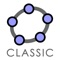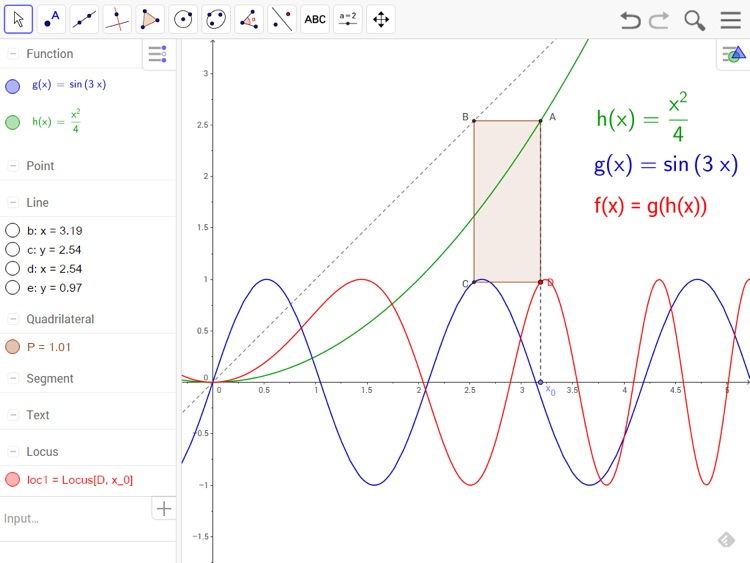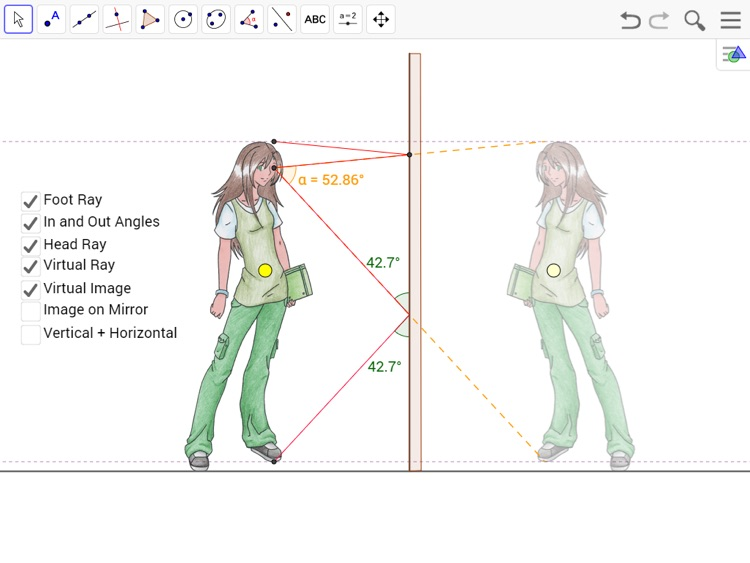## Millions of people around the world use GeoGebra Math Calculators to learn mathematics and science# GeoGebra Classic

by International GeoGebra Institute (IGI)Millions of people around the world use GeoGebra Math Calculators to learn mathematics and science. Join us: Dynamic Mathematics for everyone! Our GeoGebra Math Calculators bundle includes these apps and features:### App Details

Version
596
Rating
(60)
Size
49Mb
Genre
Education
Last updated
August 24, 2020
Release date
September 11, 2013

### App Store Description

Millions of people around the world use GeoGebra Math Calculators to learn mathematics and science. Join us: Dynamic Mathematics for everyone! Our GeoGebra Math Calculators bundle includes these apps and features:

* Graphing Calculator: plot functions with sliders and solve equations
* Geometry Calculator: create interactive geometric constructions
* 3D Calculator: graph functions, surfaces and many more 3D objects
* Spreadsheet Calc: analyze data and do statistics connected with graphing
* CAS Calculator: solve math problems with our powerful computer algebra system
* Probability Calc: visualize parameters and distributions quickly
* Exam Calculator: use our Math Calculators during tests and exams